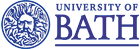## Abstract

We describe a simple but expressive calculus of sequential processes, represented as coroutines. We show that this calculus can be used to express a variety of programming language features including procedure calls, labelled jumps, integer references and stacks. We describe the operational properties of the calculus using reduction rules and equational axioms.

We describe a notion of categorical model for our calculus, and give a simple example of such a model based on a category of games and strategies. We prove full abstraction results showing that equivalence in the categorical model corresponds to observational equivalence in the calculus, and also to equivalence of \emph{evaluation trees}, which are infinitary normal forms for the calculus.

We show that our categorical model can be used to interpret the untyped lambda-calculus and use this fact to extract a sound translation of the latter into our calculus of coroutines.

• Full text (.pdf)
• Extended abstract (.pdf) (from ICALP '04).
• Slides (.pdf) from the conference presentation.
• Bibtex entry
> @article{CClong,
author = "J. Laird",
title = "A Calculus of Coroutines",
journal = "Theoretical Computer Science (ICALP '04 special issue)",
volume = 350,
pages = "275--291",
year = 2006}